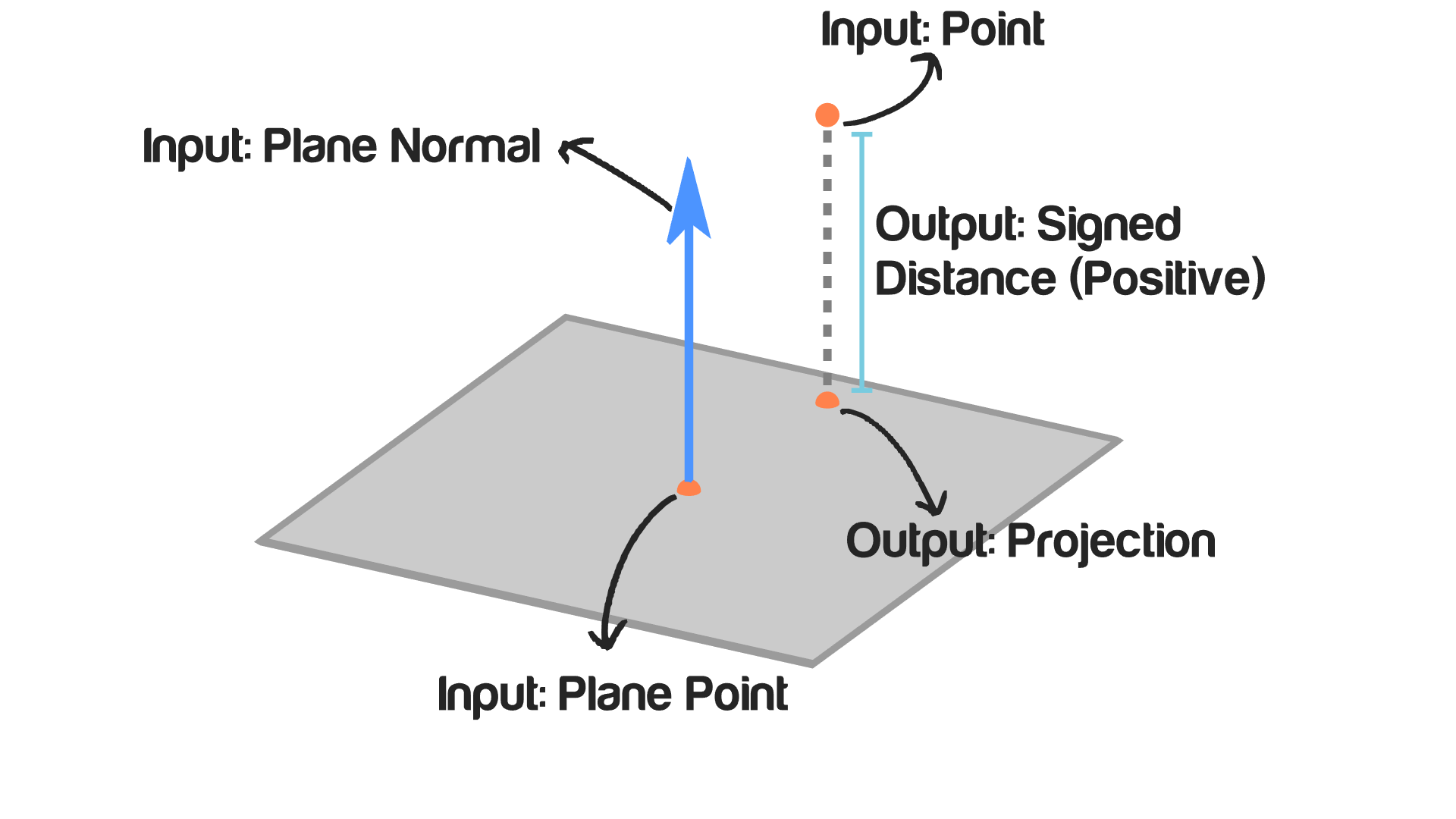# Project Point On plane

## Description

This node takes a point and a plane and returns projection of this point on that plane. The projection of a point on a plane is the closest point to the input point on the input plane.

## Illustration## Inputs

• Point - The location of the point that will be projected.
• Plane Point - A point on the plane.
• Plane Normal - The normal of the plane.

## Outputs

• Projection - The location of the closest point on the plane to the input point. Mathematically this point is the point that makes a perpendicular line to the input plane which also makes a line that is parallel to the plane normal.
• Signed Distance - It is the shortest distance between the input point and the input plane, Which is also the distance between the projected point and the input point. Although distances can’t have a negative sign, this output could have a negative sign if the point lie below the plane while positive if above.